Math Quiz For Everybody
Question 1 of 10
What is the remainder when we divide 30 by 4?
Question 2 of 10
What is the distance around a shape called?
Question 3 of 10
What is 3/4 in decimal form?
Question 4 of 10
What is the perimeter of a circle also called?
Question 5 of 10
What does the 8 mean in the number 582?
Question 6 of 10
What are the factors in this problem: 2 x 8 = 16?
Question 7 of 10
What is 3291 rounded to the nearest 1000?
Question 8 of 10
A mixed number contains what two elements?
Question 9 of 10
In the fraction 3/4, which number is the denominator?
Question 10 of 10
What does the symbol ">" mean in math?Try another one of our quizzes
World History Quiz
10 questions to test your knowledge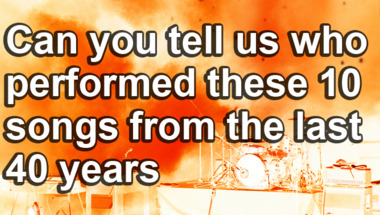General Trivia Quiz
10 impossible questionsTrivia Step Quiz
Which step do you end up on? Comment now!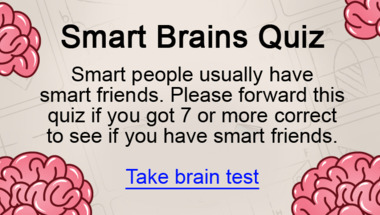1975 Song Quiz
How many of them will you answer correctly?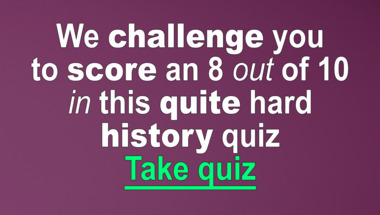Trivia Quiz
10 mixed questions for you to have fun with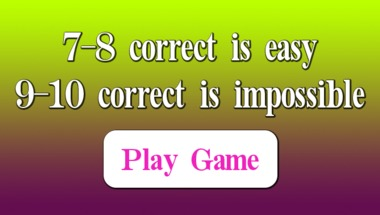The Outrageous Trivia Quiz
What do you know?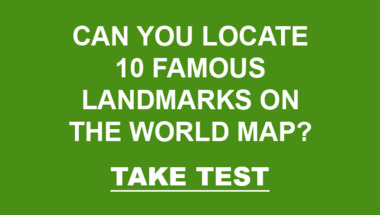World Geography Quiz
10 questions to test your knowledge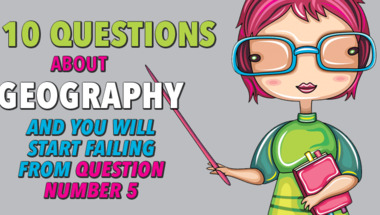World Geography Quiz
Let's test your trivia skills!Let's see how well you can spe
10 questions to test your knowledge10 questions about world histo
Share if you get more than 4 correctUnited Kingdom History Trivia
How many correct will you get?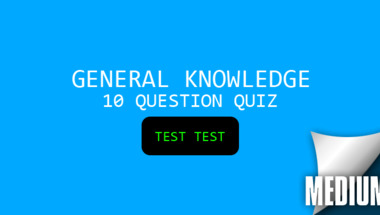General Trivia Quiz
Let's see how smart you really are!1974 Song Quiz
How many of them will you answer correctly?The Impossible Trivia Quiz
How many correct will you get?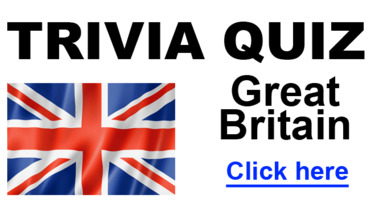1976 Song Quiz
How many of them will you answer correctly?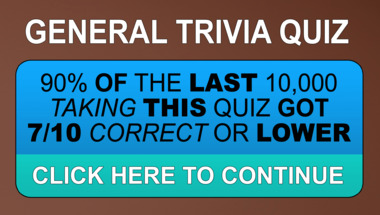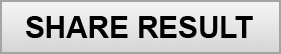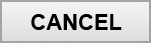Copyright 2019 - Wicked Media ApS